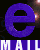The Sunyaev-Zel'dovich angular power spectrum as a probe of cosmological parameters

Eiichiro Komatsu1 and Uros Seljak2

1. Department of Astrophysical Sciences, Princeton University, Princeton, NJ 08544, USA
2. Department of Physics, Princeton University, Princeton, NJ 08544, USA

Abstract

The angular power spectrum of the SZ effect, C_l, is a powerful probe of cosmology. It is easier to detect than individual clusters in the field, is insensitive to observational selection effects and does not require a calibration between cluster mass and flux, reducing the systematic errors which dominate the cluster-counting constraints. It is also relatively insensitive to the poorly known gas physics in the cluster centre, such as cooling or (pre)heating. In this paper we derive a refined analytic prediction for C_l using the universal gas-density and temperature profile and the dark-matter halo mass function. The predicted C_l has no free parameters and fits all of the published hydrodynamic simulation results to better than a factor of two around l=3000. We find that C_l scales as (sigma_8)^7 times (Omega_b h)^2 and is almost independent of all of the other cosmological parameters. This differs from the local cluster abundance studies, which give a relation between sigma_8 and Omega_m. We also compute the covariance matrix of C_l using the halo model and find a good agreement relative to the simulations. We estimate how well we can determine sigma_8 with sampling-variance-limited observations and find that for a several-square-degree survey with 1-2 arcminute resolution one should be able to determine sigma_8 to within a few percent, with the remaining uncertainty dominated by theoretical modelling. If the recent excess of the CMB power on small scales reported by the CBI experiment is due to the SZ effect, then we find sigma_8(Omega_b h/0.035)^0.3 = 1.05 +- 0.15 at the 95% confidence level (statistical) and with a residual 10% systematic (theoretical) uncertainty.Please Contact Me !!
Mail: ekomatsu@princeton.edu